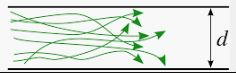# Laminar flow, Turbulence flow, Reynolds number Use of Reynolds number

Laminar flow, Turbulence flow , Reynolds number, Use of Reynolds number explained here in easy terms in detail

### Blog Content

• Flow Patterns
1. Laminar Flow
2. Turbulence flow
3. Transition flow
• Reynolds number
• Use of Reynolds number.

### Interview Questions from this blog

1. What is laminar flow, Laminar flow, Transition flow?
2. What is Reynolds number?
3. How to predict flow pattern with Reynolds number?
4. What is use of Reynolds Number?

### Flow patterns

There are two types of fluid flow pattern
1. Laminar flow pattern
2. Turbulence flow pattern
3. Transition flow pattern

#### 1. Laminar flow pattern

• Laminar flow pattern is a decent flow pattern where all layers of fluids are flowing in parallel without any disturbance.
• It following smooth path for flow. There is no swirls or eddies in flow
• Layers of fluids are flowing in straight region
• Smaller pipes with minimum flow has Laminar flow.
• Laminar flow shear stress dependent on Viscosity of fluid & Independent of density of fluids.
• Laminates is term which refers movement of adjacent fluid particles together.
• It is also known as Streamline flow or Viscous flow.Laminar flow Turbulence flow

#### Examples

1. High viscous flow at low flow ratesCredits - Quora

2.Honey dropping with gravityCredits - Quora

3. Toothpaste coming outCredits - Quora

#### Turbulence flow

• Turbulence flow is disturbed flow patterns. Where Layers has Irregular fluctuations.
• Fluids with flow rates in large diameter pipe has this turbulence flow. It creates whirlpool
• Adjacent layers fluids are crossing another fluid layers, totally distorted flow.
• Turbulent flow's shear stress depends on density of fluids.
• When flow consisting lines has more elbows, Instruments and other restrictions it makes more turbulence.
• Turbulence filters available for convert turbulence filter for flow sensors.
• Turbulence flow is also known as Chaotic flowLaminar flow

1. Water fall

#### Transition flow

It is mixed flow pattern containing both types of flow pattern in mixed.Credits - Quora

#### Reynolds number

Reynolds number is a flow pattern finding tool. we can analyse flow pattern with this formula.

Reynolds number = D * V * ρ / μ
• Re = Reynolds number
• D = Diameter of Pipe, meter
• V = Velocity of Fluids, m/s
• ρ = Density of fluid, kg/m^3
• μ = Viscosity of fluid, kg·m1·s1
If
• Reynolds number < 2300 : Laminar Flow.
• 2300 < Reynolds number < 4000 : Transition Flow.
• 4000 < Reynolds number : Turbulence flow.

### Use of Reynolds number

Reynolds number is used in
• To calculate Shell side heat transfer co efficient.
• To calculate Nusselt number in calculation of Tube side heat transfer co efficient.
The heat transfer coefficient is the heat transferred per unit area.

#### Sieder-Tate Equation for laminar flowSieder tate equation

Nu = Nusselt number
Re = Reynolds number
Pr = Preandlt number
Di = Pipe inside Diameter
µ = Fluid Viscosity of fluid bulk temperature
µW = Fluid Viscosity at pipe wall temperature

#### Dittus-Bolter Equation for Turbulence flowDittus bolter equation for tube side heat transfer co efficient in turbulence flow

Nu = Nusselt number
Re = Reynolds number
Pr = Preandlt number
µ = Fluid Viscosity of fluid bulk temperature
µW = Fluid Viscosity at pipe wall temperature